# Recursion and stack

## Introduction

In this article, we are going to learn a significant topic in programming, i.e., recursion. It is very beneficial for solving a task that can be divided into several smaller functions of the same type with ease.

So, without any further ado, let's get started!

## What is recursion

When a function calls itself, it is called a recursive function. A recursive function is an alternative for loops in logic that are expressible in the form of themselves. It is an elegant way of solving different problems. It's advantageous when you need to break down a complicated task into several smaller ones.Source: Unsplash

To understand recursion, we will first look at the iterative approach of finding factorial of a number. Then we will see how we can calculate factorial by using the recursive function:

fact(1)=1

fact(2)=2

fact(3)=6

fact(4)=24

fact(5)=120

## Iterative method

Here we will find the factorial of a number using the for loop:

``````function fact(n) {
for (let i = 1; i<n; i++) {
ans *= i;
}
}
fact(9);``````

## Recursive approach

Now, we will find the factorial using the recursive method. It can be divided into a base case and the logic part. The base case is very important because we can’t come out of the function, leading to the infinite recursive call. Hence, stack overflow will occur.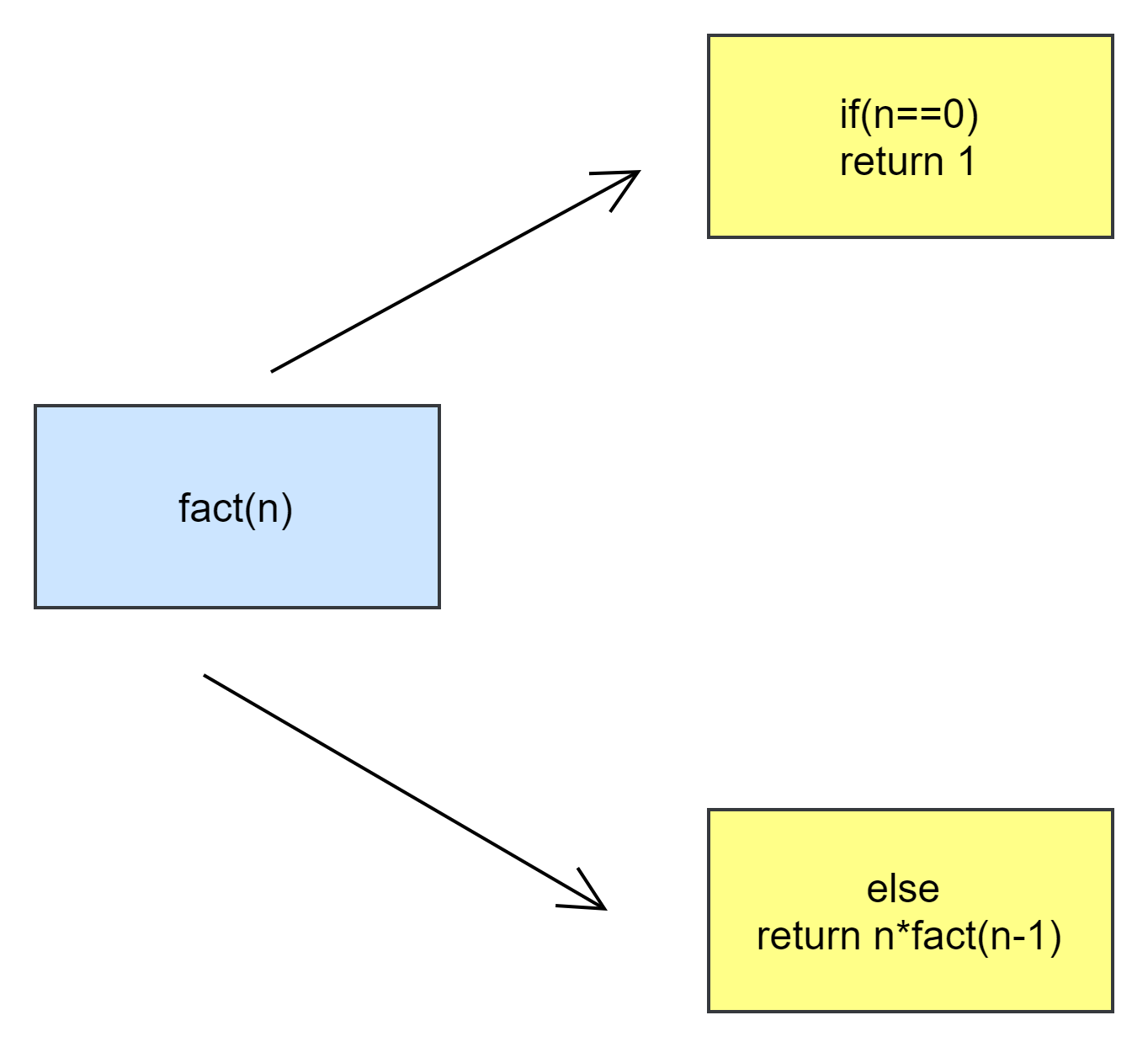In this picture above,upper block (i.e. if(n==0)) acts as a base case. The lower block is the logic part of the function.

The code for the recursive factorial function is:

``````let fact = function(n) {
if(n == 0) {
return 1
} else {
return n * fact(n - 1); //function calling itself
}
}
console.log(fact(9));``````

## The Execution Context and Stack

The execution context is an internal data structure that contains information about a function's execution, such as the current control flow, current variables, the value of this, and a few other internal details. There is only one execution context associated with each function call.

Let's see how recursive function calls work. The following happens when a function makes a nested call:

• The current function is put on hold.
• A special data structure called the execution context stack is used to remember its associated execution context.
• The nested call executes.
• The old execution context is obtained from the stack after it finishes, and the outer function is resumed.

Let's take a look at what happens when the fact(5) call is made.

``````let fact = function(n) {
if(n == 0) {
return 1
} else {
return n * fact(n - 1); //function calling itself
}
}
console.log(fact(5));``````

Context: { n: 5, at line 1 } factorial(5) :  The execution context initially stores the n = 5 variable. The execution flow is at the first function’s line. It will go to the else block (logic part) as n is not equal to 0. Hence it will make a recursive call for 4 (i.e., n-1).

The stack will look like this: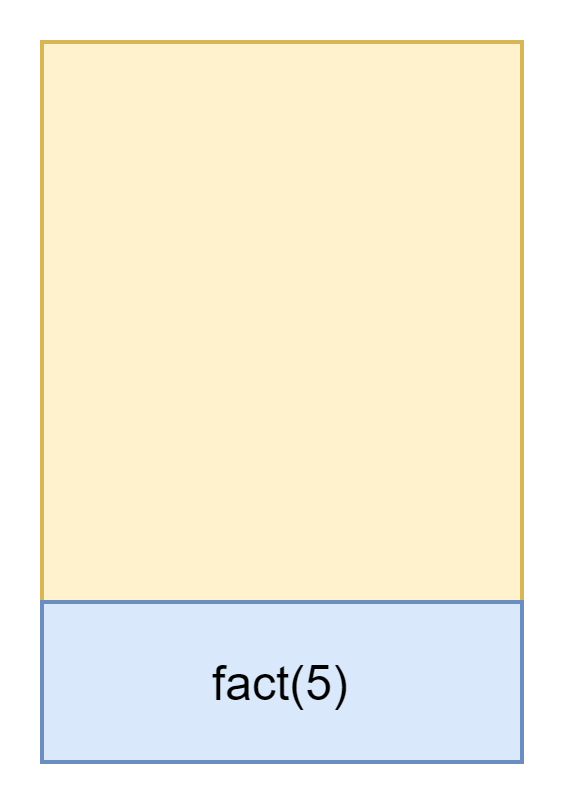Context: { n: 4, at line 2 } factorial(4): JavaScript remembers the current execution context in the execution context stack when making a nested call. The execution context will store the n = 4 variable. The execution flow is at the second function’s line. It will go to the else block (logic part) as n is not equal to 0. Hence it will make a recursive call for 3 (i.e., n-1).

The stack will look like this: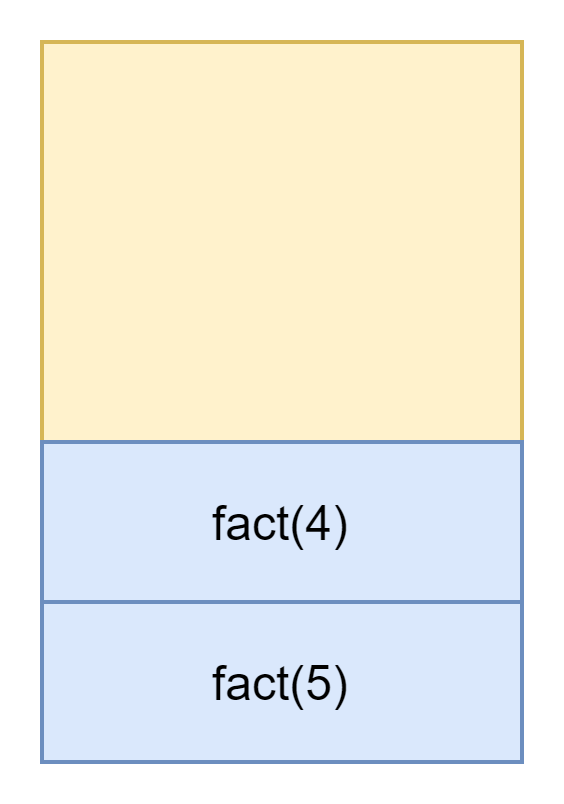Context: { n: 3, at line 3 } factorial(3): The execution context will store the n = 3 variable at the top of the stack. The execution flow is at the third function’s line. It will go to the else block (logic part) as n is not equal to 0. Hence it will make a recursive call for 2 (i.e., n-1).

The stack will look like this: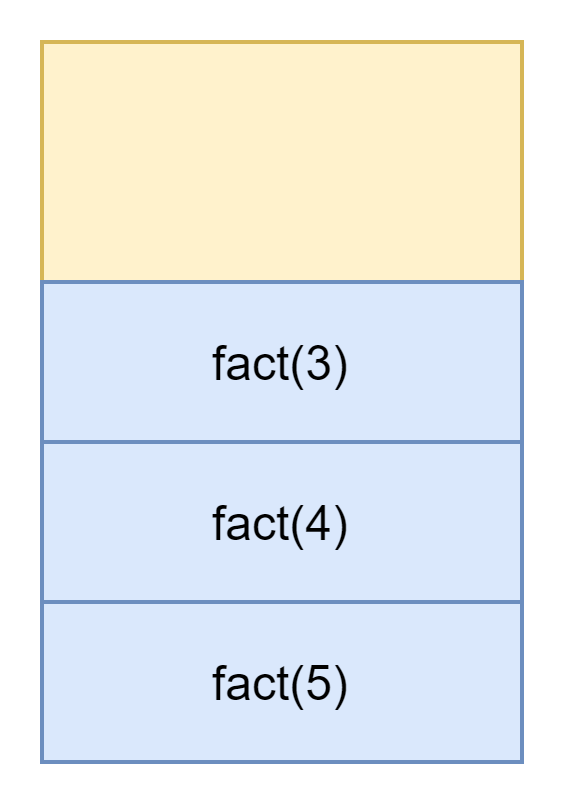Context: { n: 2, at line 4 } factorial(2): The execution context will store the n = 2 variable. The execution flow is at the fourth function’s line. It will go to the else block (logic part) as n is not equal to 0. Hence it will make a recursive call for 1 (i.e., n-1).

The stack will look like this: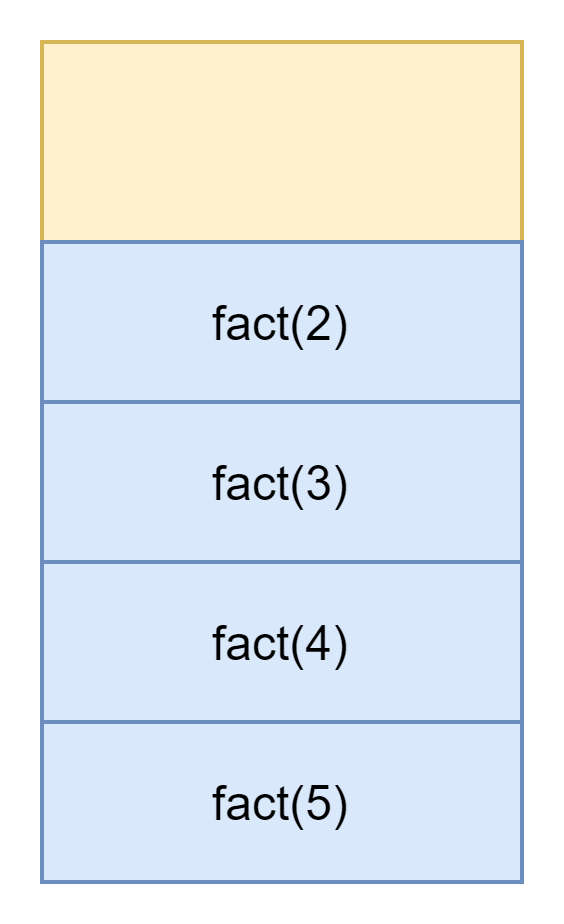Context: { n: 1, at line 5 } factorial(1): The execution context will store the n = 1 variable. The execution flow is at the fifth function’s line. It will go to the else block (logic part) as n is not equal to 0. Hence it will make a recursive call for 0 (i.e., n-1).

The stack will look like this: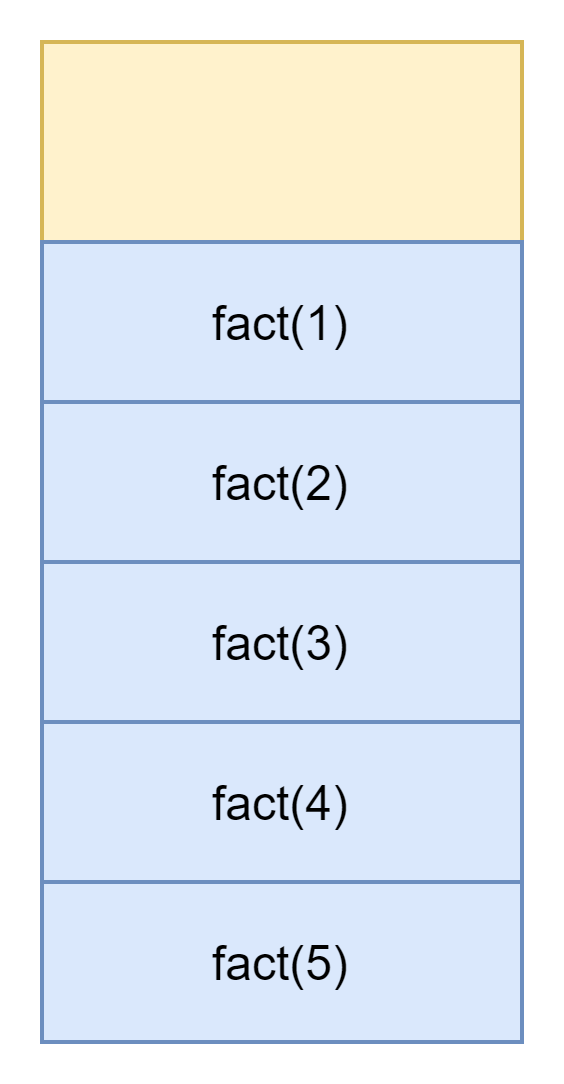After this, fact(1) will call for 0 (i.e., n-1), which is the base case of the recursive function, then the following will take place:

1. It will return 1 to its parent call, i.e., fact(1).
2. Then fact(1) will return 1 to its parent call, i.e., fact(2).
3. Then fact(2) will return 2 to its parent call, i.e., fact(3).
4. Then fact(3) will return 6 to its parent call, i.e., fact(4).
5. Then fact(4) will return 24 to its parent call, i.e., fact(5).
6. Now fact(5) will return the final answer of the function, i.e., 120.

The diagram below is a pictorial representation of the recursive function.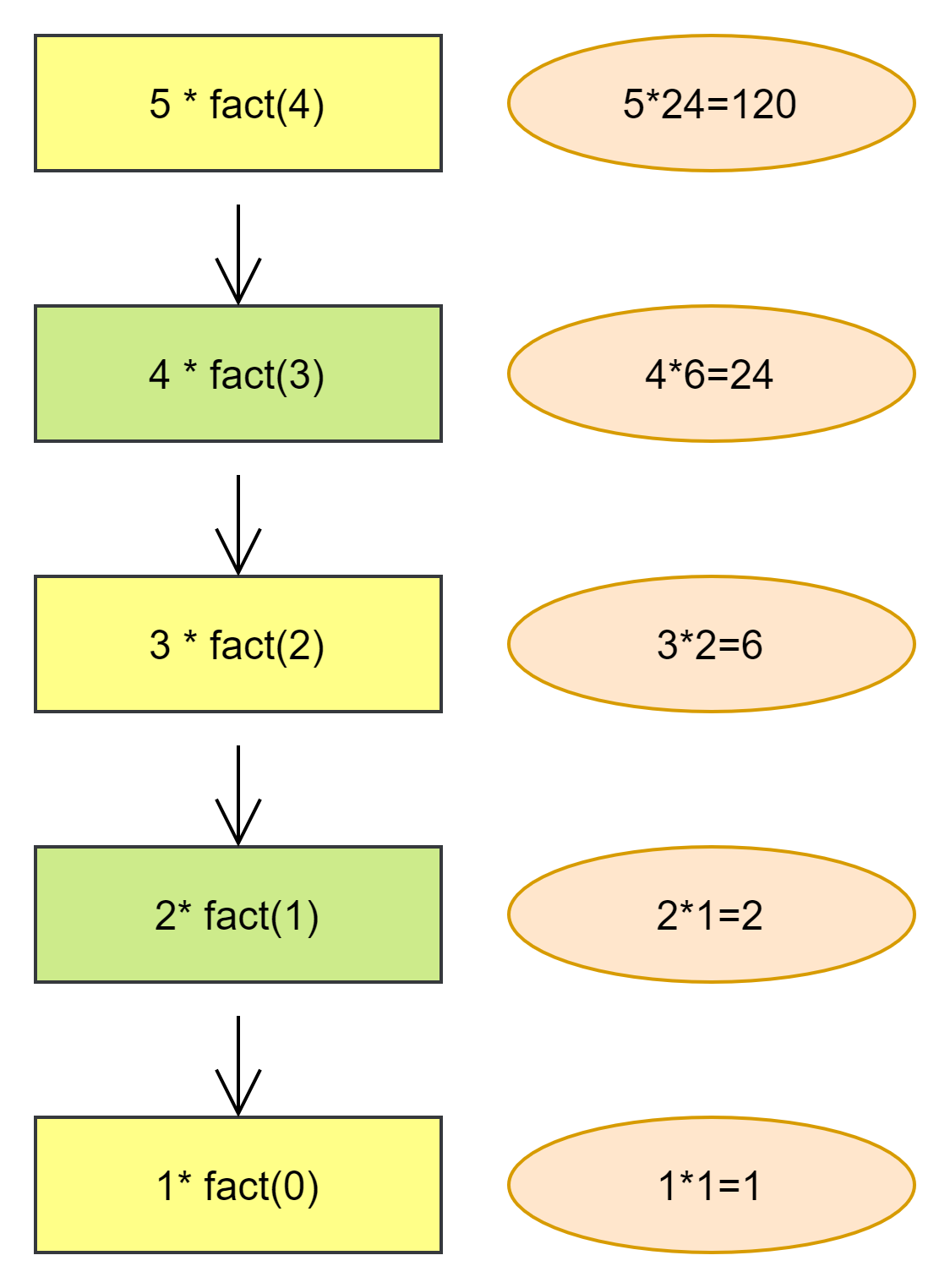Q1) What are the drawbacks of recursion?

Answer: The drawbacks of recursion are as follows:

• Sometimes recursive functions are slower than non-recursive functions.
• It's possible that storing intermediate results on the system stacks will take up a lot of memory.
• The code is difficult to analyze.
• It is not more efficient due to its space and time complexity.

Q2) What is the significance of recursion?

Answer: In programming, recursive thinking is essential. It aids in the decomposition of large problems into smaller ones. The recursive solution is frequently easier to read than the iterative one.

Q3) What is the fundamental rule of recursion?

Answer: Three important rules must be followed by all recursive algorithms:

1. A recursive algorithm must call itself.
2. A base case is required for a recursive algorithm.
3. The state of a recursive algorithm must be changed in order for it to return to the base case.

## Key Takeaways

To learn more about stacks in Java, you can visit this amazing article, Stack class in Java Collection Framework. Similarly in Python and C++.

If you want to learn more about recursion, this is the place to go. You can access a variety of recursion problems and concepts here.